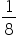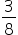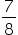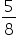Mathematics
Easy

Question

# If a coin is tossed thrice, then the probability of getting head twice is_________________.

##Hint:

## The correct answer is:### Here, a coin is tossed thrice  are : (H, H, H) , ( H, H,T) , ( H, T, H) , ( T, H, H) , ( T, T, T) , ( T, T, H) , ( T, H, T) , ( H, T, T) .Total number of outcomes = 8Number of favourable outcome = Number of outcomes with 2 tails = 3Therefore,  P ( getting head twice) = 3/8Hence, the correct option is (b).

0 ≤ P(E) ≤ 1#### With Turito Foundation.#### Get an Expert Advice From Turito.